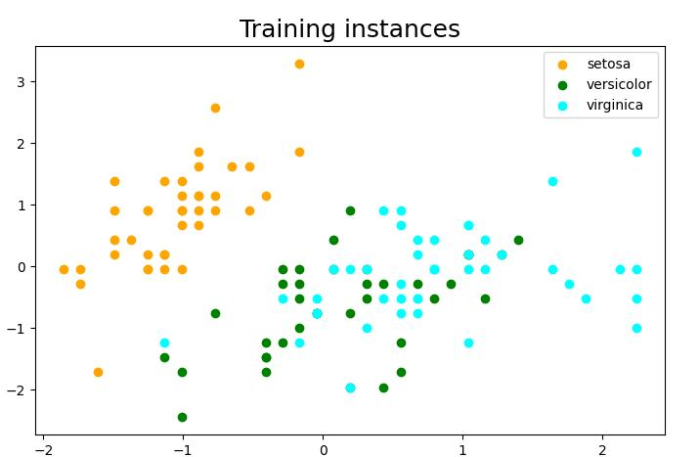# How to implement linear classification with Python Scikit-learn?

Linear classification is one of the simplest machine learning problems. To implement linear classification, we will be using sklearn’s SGD (Stochastic Gradient Descent) classifier to predict the Iris flower species.

## Steps

You can follow the below given steps to implement linear classification with Python Scikit-learn −

Step 1 − First import the necessary packages scikit-learn, NumPy, and matplotlib

Step 2 − Load the dataset and build a training and testing dataset out of it.

Step 3 − Plot the training instances using matplotlib. Although this step is optional, it is good practice to plot the instances for more clarity.

Step 4 − Create object of the SGD classifier, initialize its parameters and train the model using fit() method.

Step 5 − Evaluate the result by using the metrics package of Python Scikit-learn library.

## Example

Let’s check the example below in which we will be predicting Iris flower species using its two features namely sepal width and sepal length −

# Import required libraries
import sklearn
import numpy as np
import matplotlib.pyplot as plt
# %matplotlib inline

from sklearn import datasets
X_data, y_data = iris.data, iris.target

# Print iris data shape
print ("Original Dataset Shape:",X_data.shape, y_data.shape)

# Dividing dataset into training and testing dataset and standarized the features
from sklearn.model_selection import train_test_split
from sklearn.preprocessing import StandardScaler

# Getting the Iris dataset with only the first two attributes
X, y = X_data[:,:2], y_data

# Split the dataset into a training and a testing set(20 percent)
X_train, X_test, y_train, y_test = train_test_split(X, y, test_size=0.20, random_state=1)
print ("\nTesting Dataset Shape:", X_train.shape, y_train.shape)

# Standarize the features
scaler = StandardScaler().fit(X_train)
X_train = scaler.transform(X_train)
X_test = scaler.transform(X_test)

# Plot the dataset
# Set the figure size
plt.figure(figsize=(7.16, 3.50))
plt.title('Training instances', size ='18')
colors = ['orange', 'green', 'cyan']
for i in range(len(colors)):
px = X_train[:, 0][y_train == i]
py = X_train[:, 1][y_train == i]
plt.scatter(px, py, c=colors[i])

plt.legend(iris.target_names)
plt.xlabel('Sepal length')
plt.ylabel('Sepal width')
plt.show()

# create the linear model SGDclassifier
from sklearn.linear_model import SGDClassifier
linear_clf = SGDClassifier()

# Train the classifier using fit() function
linear_clf.fit(X_train, y_train)

# Print the learned coeficients
print ("\nThe coefficients of the linear boundary are:", linear_clf.coef_)
print ("\nThe point of intersection of the line are:",linear_clf.intercept_)

# Evaluate the result
from sklearn import metrics
y_train_pred = linear_clf.predict(X_train)
print ("\nThe Accuracy of our classifier is:", metrics.accuracy_score(y_train, y_train_pred)*100)


## Output

It will produce the following output

Original Dataset Shape: (150, 4) (150,)

Testing Dataset Shape: (120, 2) (120,)

The coefficients of the linear boundary are: [[-28.85486061 13.42772422]
[ 2.54806641 -5.04803702]
[ 7.03088805 -0.73391906]]

The point of intersection of the line are: [-19.61738307 -3.54055412 -0.35387805]


The Accuracy of our classifier is: 76.66666666666667